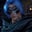Related Tags

javascript
communitycreator

# How to swap variables using destructuring in JavaScriptGutha Vamsi Krishna

In this shot, we will learn how to swap variables using destructuring assignment in JavaScript. We will start with a problem statement, followed by an example.

### Problem

Swap the two given variables using the JavaScript destructuring feature.

#### Example

Input: x=5, y=10

Output: x=10, y=5

### Solution

We will start solving this problem by looking at the destructuring concept.

JavaScript’s destructuring is used to unpack the values present in arrays or object properties into distinct variables.

//declare and initialize an array
let arr = ["apple","orange"]

//destructing the arrary arr
let [a,b] = arr

//print a and b values
console.log(a,b)

### Explanation

In the above code snippet:

• Line 2: We declare and initialize an array arr.
• Line 5: Using the destructuring assignment, we unpack the values of arr and store them in variables a and b.
• Line 8: We print the values to the console.

### Swap the variables

//given variables
let x = 5;
let y = 10;

console.log("Before:")
console.log(x,y)

//assign them as array to temp
let temp = [y,x];

//destructure
[x,y] = temp

console.log("After:")
console.log(x,y)



### Explanation

In the above code snippet:

• Line 2 and 3: We declare and initialize variables x and y.
• Line 9: We pass x and y as an array in reverse order and assign it to temp.
• Line 12: We destructure the array temp and assign it to x and y.

RELATED TAGS

javascript
communitycreator

CONTRIBUTORGutha Vamsi Krishna
RELATED COURSES

View all Courses

Keep Exploring

Learn in-demand tech skills in half the time